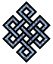#fail2ban bad ip database: ip 18.236.169.41

### | ip database | live view | stats | report | help | api key:

 ip: 18.236.169.41 hostname: ec2-18-236-169-41.us-west-2.compute.amazonaws.com country:[US] United States first reported: 20.11.2018 22:39.29 GMT+0200 last reported: 25.11.2018 13:14.24 GMT+0200 time period: 4d 14h 34m 55s total reports: 11 reported by: 2 host(s) filter(s): ssh (6) ssh (5) tor exit node no badips.com db Lookup## port scan of '18.236.169.41':

[-hide]
```# Nmap 6.40 scan initiated Tue Nov 20 22:40:03 2018 as: /usr/bin/nmap -sU -sS -O 18.236.169.41
Nmap scan report for ec2-18-236-169-41.us-west-2.compute.amazonaws.com (18.236.169.41)
Host is up (0.16s latency).
Not shown: 1000 open|filtered ports, 993 filtered ports
PORT     STATE  SERVICE
22/tcp   open   ssh
25/tcp   closed smtp
80/tcp   open   http
443/tcp  closed https
587/tcp  closed submission
3306/tcp closed mysql
8083/tcp open   us-srv
No exact OS matches for host (If you know what OS is running on it, see http://nmap.org/submit/ ).
TCP/IP fingerprint:
OS:SCAN(V=6.40%E=4%D=11/20%OT=22%CT=25%CU=%PV=N%G=Y%TM=5BF47F75%P=x86_64-pc
OS:-linux-gnu)SEQ(SP=100%GCD=1%ISR=106%TI=Z%CI=I%TS=8)SEQ(SP=100%GCD=1%ISR=
OS:106%TI=Z%TS=8)OPS(O1=M5B4ST11NW7%O2=M5B4ST11NW7%O3=M5B4NNT11NW7%O4=M5B4S
OS:T11NW7%O5=M5B4ST11NW7%O6=M5B4ST11)WIN(W1=68DF%W2=68DF%W3=68DF%W4=68DF%W5
OS:=68DF%W6=68DF)ECN(R=Y%DF=Y%TG=40%W=6903%O=M5B4NNSNW7%CC=Y%Q=)T1(R=Y%DF=Y
OS:%TG=40%S=O%A=S+%F=AS%RD=0%Q=)T2(R=N)T3(R=N)T4(R=Y%DF=Y%TG=40%W=0%S=A%A=Z
OS:%F=R%O=%RD=0%Q=)T5(R=Y%DF=Y%TG=40%W=0%S=Z%A=S+%F=AR%O=%RD=0%Q=)T6(R=Y%DF
OS:=Y%TG=40%W=0%S=A%A=Z%F=R%O=%RD=0%Q=)T7(R=Y%DF=Y%TG=40%W=0%S=Z%A=S+%F=AR%
OS:O=%RD=0%Q=)U1(R=N)IE(R=N)

OS detection performed. Please report any incorrect results at http://nmap.org/submit/ .
# Nmap done at Tue Nov 20 22:41:09 2018 -- 1 IP address (1 host up) scanned in 66.65 seconds
```
```Σ = 41 | Δt = 0.0041229724884033s
```